### Work and Energy - Solutions

CBSE class IX Science

NCERT Solutions
Chapter - 11
Work and Energy

(Page No.148)

1. A force of 7 N acts on an object. The displacement is, say 8 m, in the direction of the force. Let us take it that the force acts on the object through the displacement. What is the work done in this case?

Ans. Since Work (W)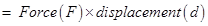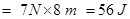(Page No.149)

1. When do we say that work is done?

Ans. Work is done when a force acts on an object and displacement occurs in the direction of force.

2. Write an expression for the work done when a force is acting on an object in the direction of its displacement.

Ans. Work = Force x displacement
W = F  x d

3. Define 1 J of work.

Ans. 1J is that work which is done when a force of 1N is applied on an object and it moves a distance of 1m in the direction of force.

4. A pair of bullocks exerts a force of 140 N on a plough. The field being ploughed is 15 m long. How much work is done in ploughing the length of the field?

Ans. Work done W = F x d
= 140 N x 15 m = 2100 J

(Page No.152)

1. What is the kinetic energy of an object?

Ans. The kinetic energy of an object is a kind of mechanical energy and it is present in that object due to its state of motion(movement).

2. Write an expression for the kinetic energy of an object.

Ans. Kinetic energy =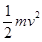where, m = mass of the object, v = velocity of the object(motion)

3. The kinetic energy of an object of mass, m moving with a velocity of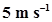is 25 J. What will be its kinetic energy when its velocity is doubled? What will be its kinetic energy when its velocity is increased three times?

Ans. K.E. of the object =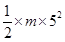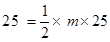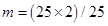= 2 kg

If velocity is doubled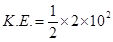= 200/2 = 100 J i.e. K.E. will become four times

If velocity is increased three times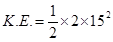= 225 J i.e. K.E. will become nine times

(Page No.156)

1. What is power?

Ans. 1. Power is the rate of work done. It is denoted by P.

power = work/time

P = W / t

2. Define 1 Watt of power.

Ans. If an agent works at the rate of 1 J/s then the power of that agent is 1 Watt (where Watt is the unit of power).

3. A lamp consumes 1000 J of electrical energy in 10 s. What is its power?

Ans. P = W/t = 1000J/10s = 100 W

4. Define average power.

Ans. An agent may not be able to perform the same amount of work in a given period of time always. In other words, the power of that agent may vary with time. Hence we can take average power in such situations. Average power is defined as the average amount of work done by a body per unit time (i.e. total energy consumed divided by total time).

(Chapter – end)

1. Look at the activities listed below. Reason out whether or not work is done in the light of your understanding of the term ‘work’.

• Suma is swimming in a pond.

• A donkey is carrying a load on its back.

• A windmill is lifting water from a well.

• A green plant is carrying out photosynthesis.

• An engine is pulling a train.

• Food grains are getting dried in the sun.

• A sailboat is moving due to wind energy.

Ans. Work will be done if a force acts on an object and displacement occurs in the direction of force. According to this explanation work is done in following activities:

• Suma is swimming in a pond.

• A donkey is carrying a load on its back.

• A windmill is lifting water from a well.

• An engine is pulling a train.

• A sailboat is moving due to wind energy.

2. An object was thrown at a certain angle to the ground moves in a curved path and falls back to the ground. The initial and the final points of the path of the object lying on the same horizontal line. What is the work done by the force of gravity on the object?

Ans.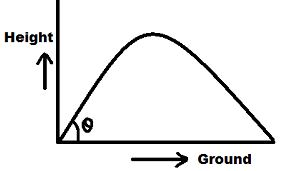For calculating work done there must be displacement but since in this example vertical displacement is zero (as initial and final points lie on the same horizontal line) so the work done by force of gravity is zero.

3. A battery lights a bulb. Describe the energy changes involved in the process.

Ans. Chemical energy of the battery is changed into heat energy and light energy.

4. A Certain force acting on a 20 kg mass changes its velocity from 5 ms -1 to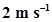. Calculate the work done by the force.

Ans. Work done by the force = change in kinetic energy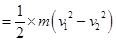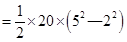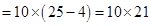= 210 J

5. A mass of 10 kg is at a point A on a table. It is moved to a point B. If the line joining A and B is horizontal, what is the work done on the object by the gravitational force? Explain your answer.

Ans. Since work done on the object by the gravitational force depends upon the change in the vertical height of the object. The vertical height of the object is not changing as the line joining A and B is horizontal at the same height hence the work done is zero.

6. The potential energy of a freely falling object decreases progressively. Does this violate the law of conservation of energy? Why?

Ans. It doesn’t violate the law of conservation of energy because the potential energy of a freely falling object decreases progressively since it keeps changing into kinetic energy of the free falling object, therefore, the total energy of the object remains conserved.

7. What are the various energy transformations that occur when you are riding a bicycle?

Ans. It is the transformation of our muscular energy into mechanical energy to ride the bicycle.

8. Does the transfer of energy take place when you push a huge rock with all you might and fail to move it? Where is the energy you spend going?

Ans. While we push a huge rock with all our might(power) but fail to move it no energy transfer occurs as cellular energy simply wastes out in muscle contraction and relaxation even heat generation(sweating).

9. A certain household has consumed 250 units of energy during a month. How much energy is this in joules?

Ans. Energy consumed by a certain household = 250 kWh

since 1 kWh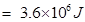therefore 250 kWh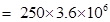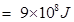10. An object of mass 40 kg is raised to a height of 5 m above the ground. What is its potential energy? If the object is allowed to fall, find its kinetic energy when it is half way down.

Ans. Potential energy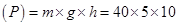= 2000 J

When the object is half way down the height of the object is = 2.5 m

initial velocity (u) = 0 (thrown from ground/rest)

since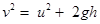= 0 + 2 x 10 x 2.5
= 50
Kinetic energy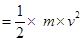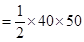= 1000 J

11. What is the work done by the force of gravity on a satellite moving around the earth? Justify your answer.

Ans. The satellite is moving on around the earth, displacement of the object is perpendicular to the direction of force.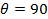0.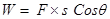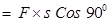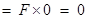Therefore, work done is zero.

12. Can there be displacement of an object in the absence of any force acting on it? Think. Discuss this question with your friends and teacher.

Ans. No. there can not be any displacement without any force involved.

13. A person holds a bundle of hay over his head for 30 minutes and gets tired. Has he done some work or not? Justify your answer.

Ans. When a person holds a bundle of hay over his head for 30 minutes, he applies force in the upward direction, against the gravity, so displacement is in the direction of applied force.Hence angle between force and displacement is zero.

Here, F=mg and S=h(height to which the person holds the hay)

we know

$W=F×S\mathrm{Cos}\theta$

$F×S\mathrm{Cos}{0}^{\circ }$

$=F×S$

=$mg×h$

W=mgh

14. An electric heater is rated 1500 W. How much energy does it use in 10 hours?

Ans. Electric heater’s power (p) = 1500 W = 1.5 kW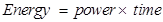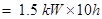= 15 kWh

15. Illustrate the law of conservation of energy by discussing the energy changes which occur when we draw a pendulum bob to one side and allow it to oscillate. Why does the Bob eventually come to rest? What happens to its energy eventually? Is it a violation of the law of conservation of energy?

Ans. In the case of pendulum energy in the Bob at any instant of time, can be either potential energy (P.E) or kinetic energy (K.E) or a mixture of both but its total energy at any instant of time remains constant. This can be illustrated by below illustration in the figure.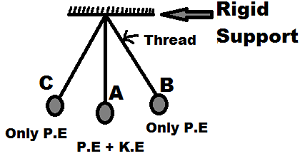In case of pendulum energy in the Bob at any instant of time
when the pendulum Bob is at position B.It has only potential energy(P.E) and no kinetic energy(K.E)
As the Bob starts moving from position B to position A potential energy(P.E) decreases but Kinetic Energy(K.E) increases
when the Bob reaches position A, there is only kinetic energy(K.e) but no potential energy(P.E)
As Bob moves from position A to position C, potential energy(p.E) increases but kinetic energy decreases.
when Bob reaches position C, Bob stops for a very small instant of time, At that time, Bob has only potential energy but no kinetic energy.

The Bob eventually comes to rest due to the frictional force offered by the air and the rigid support holding the thread.

It is not a violation of the law of conservation of energy since mechanical energy can get converted into another form of energy which cannot be utilized for useful work. This loss of energy is called dissipation of energy.

16. An object of mass, is moving with a constant velocity, v. How much work should be done on the object in order to bring the object to rest?

Ans. In order to bring the object to rest the work done must be equal to the kinetic energy of the moving object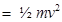17. Calculate the work required to be done to stop a car of 1500 kg moving at a velocity of 60 km/h?

Ans. Initial velocity of the car (u) = 60 km/h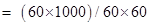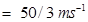Final velocity (v) = 0 (object has to be stopped)

Initial kinetic energy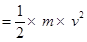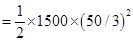= 208333.30 J

Final kinetic energy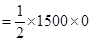= 0

Therefore, work done = change in kinetic energy = 208333.30 ― 0 = 208333.30 J

18. In each of the following a force, is acting on an object of mass, m. The direction of displacement is from west to east shown by the longer arrow. Observe the diagrams carefully and state whether the work done by the force is negative, positive or zero.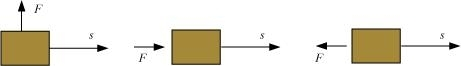Ans. (i) Since in this diagram displacement is perpendicular to the direction of force, so work done is zero.

(ii) Since in this diagram displacement is in the direction of force, so work done is positive.

(iii) Since in this diagram displacement is in the opposite direction of the force applied hence work done is negative.

19. Soni says that the acceleration of an object could be zero even when several forces are acting on it. Do you agree with her? Why?

Ans. Yes, we agree with her statement. Because when many balanced forces acting on the object its displacement becomes zero.

20. Find the energy in kW h consumed in 10 hours by four devices of power 500 W each.

Ans. Since Energy = power x time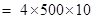= 20000 Wh = 20 kWh

21. A freely falling object eventually stops on reaching the ground. What happens to its kinetic energy?

Ans. A free falling object eventually stops on reaching the ground since on striking the ground its kinetic energy is transmitted to the ground.